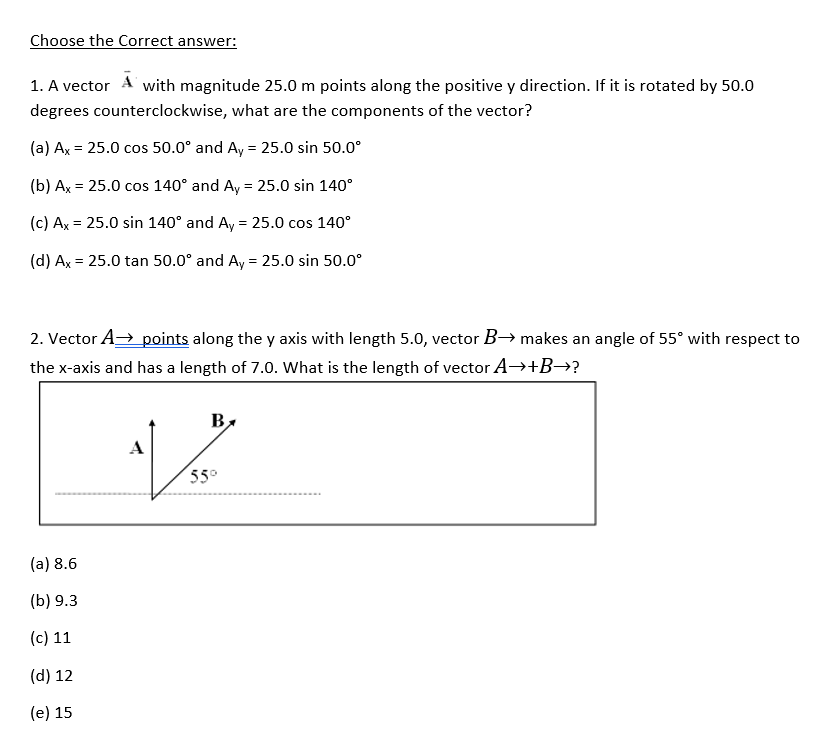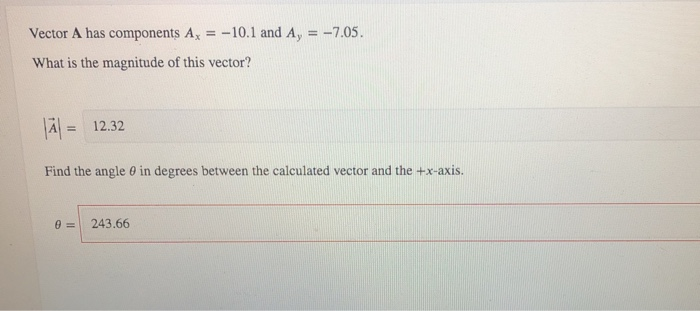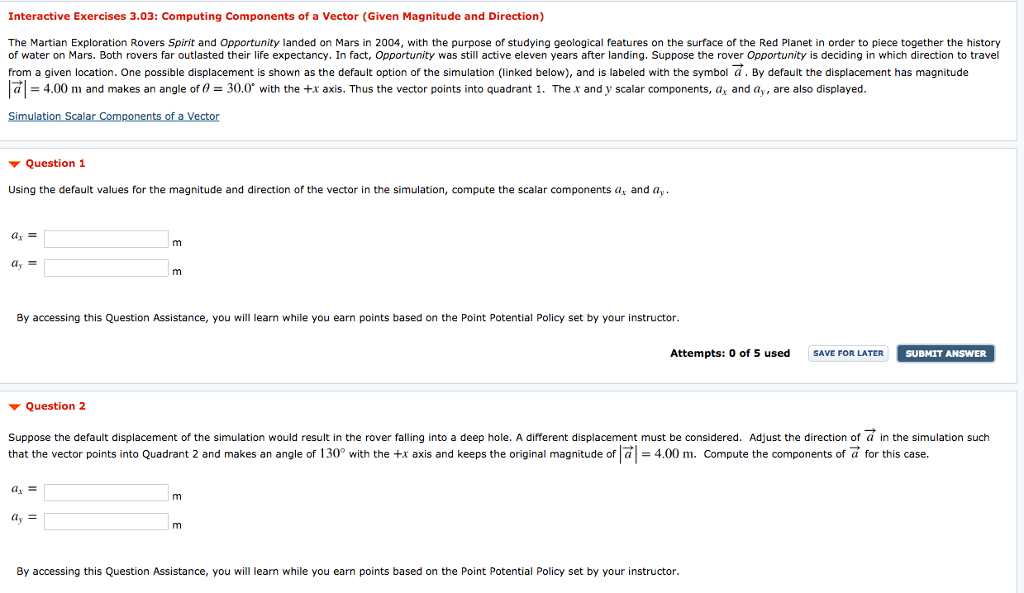# Given the length of the vector and angle, determine the components of the vector. Magnitude (length)...

Given the length of the vector and angle, determine the components of the vector. Magnitude (length) of A = 5.0990 Angle vector A makes with respect to the x-axis = 168.6901 degrees

1. Ax =

2. Ay =

here,

magnitude of vector A , |A| = 5.099 units

theta = 168.6901 degree

the x-compoenent of vector , Ax = A * cos(theta)

Ax = 5.099 * cos(168.6901)

Ax = - 5 units

the y-component of vector , Ay = A * sin(theta)

Ay = 5.099 * sin(168.6901)

Ay = 1 unit

##### Add Answer of: Given the length of the vector and angle, determine the components of the vector. Magnitude (length)...
Similar Homework Help Questions
• ### Choose the Correct answer: 1. A vector A with magnitude 25.0 m points along the positive...Choose the Correct answer: 1. A vector A with magnitude 25.0 m points along the positive y direction. If it is rotated by 50.0 degrees counterclockwise, what are the components of the vector? (a) Ax 25.0 cos 50.0° and Ay 25.0 sin 50.0 (b) Ax 25.0 cos 140° and Ay 25.0 sin 140 (c) Ax 25.0 sin 140° and Ay 25.0 cos 140 (d) Ax- 25.0 tan 50.0° and Ay 25.0 sin 50.0° 2. Vector A→points along the y axis...

• ### Consider the two vectors, A and B, for which the length and angle are given below....

Consider the two vectors, A and B, for which the length and angle are given below. What would be the x and y components of the the vector sum C = A + B? length of A = 4.472, angle A makes with respect to x-axis = 153.435 degrees length of B = 3.606, angle C makes with respect to x-axis = 56.310 degrees Cx =   Cy=

• ### Vector A has components A-10.1 and Ay 7.05 What is the magnitude of this vector? A...Vector A has components A-10.1 and Ay 7.05 What is the magnitude of this vector? A 12.32 Find the angle θ in degrees between the calculated vector and the +x-axis. θ | 243.66

• ### Vector A⃗ has components Ax = 1.24 cm , Ay = 2.36 cm ; vector B⃗...

Vector A⃗ has components Ax = 1.24 cm , Ay = 2.36 cm ; vector B⃗ has components Bx = 4.03 cm , By = -3.74 cm . Find the components of the vector sum A⃗ +B⃗ Find the magnitude of the vector sum A⃗ +B⃗ Find the counterclockwise angle the vector sum A⃗ +B⃗ makes with the +x axis, in interval [0,360]∘. Find the components of the vector difference B⃗ −A⃗ . Find the magnitude of the vector difference...

• ### Interactive Exercises 3.03: Computing Components of a Vector (Given Magnitude and Direction) The Martian Exploration Rovers...Interactive Exercises 3.03: Computing Components of a Vector (Given Magnitude and Direction) The Martian Exploration Rovers Spirit and Opportunity landed on Mars in 2004, with the purpose of studying geological features on the surface of the Red Planet in order to piece together the history of water on Mars. Both rovers far outlasted their life expectancy. In fact, Opportunity was still active eleven years after landing. Suppose the rover Opportunity is deciding in which direction to travel from a given...

• ### Vector A has components Ax = 1.32 cm , Ay = 2.43 cm ; vector B...

Vector A has components Ax = 1.32 cm , Ay = 2.43 cm ; vector B has components Bx = 4.19 cm , By = -3.65 cm . A) Find the components of the vector sum A + B. B) Find the magnitude of the vector sum A +B . C) Find the counterclockwise angle the vector sum A +B makes with the +x axis. Please give your answer in the interval [0,360] D )Find the components of the vector...

• ### In each of the cases that follow, the components of a vector A⃗  are given. Use trigonometry...

In each of the cases that follow, the components of a vector A⃗  are given. Use trigonometry to find the magnitude of that vector and the counterclockwise angle it makes with the +xaxis. Ax = 3.00 m , Ay = 8.00 m Please give your answer in the interval [0,360]∘.

• ### find the magnitude

Find the magnitude of the vector A represented by the pair of components Ax= 7.80 km , Ay= -2.60 kmandFind the direction of the vector . Let the direction of a vector be the angle that the vector makes with the +x-axis, measured counterclockwise from that axis

• ### Vector A⃗ has components Ax= 1.80 cm , Ay= 2.25 cm ; vector B⃗ has components...

Vector A⃗ has components Ax= 1.80 cm , Ay= 2.25 cm ; vector B⃗ has components Bx= 4.10 cm , By= -3.50 cm . Find the direction of A⃗ +B⃗ . (Let the direction be the angle that the vector makes with the +x-axis, measured counterclockwise from that axis.) please show calculations

• ### A vector A has components Ax = −3.50 m and Ay = 5.00 m. Find the...

A vector A has components Ax = −3.50 m and Ay = 5.00 m. Find the magnitude (in m) and the direction (in degrees counterclockwise from the +x-axis) of the vector.

Need Online Homework Help?